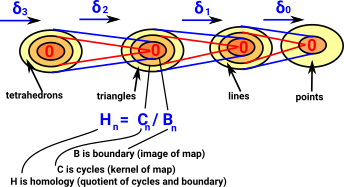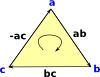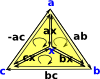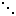# Homology

## Algebraic Topology

As introduced on page here: In two dimensions it is relatively easy to determine if two spaces are topologically equivalent (homeomorphic). We can check if they:

• are connected in the same way.
• have the same number of holes.

However, when we scale up to higher dimensions this does not work and it can become impossible to determine homeomorphism. There are methods which will, at least, allow us to prove more formally when topological objects are not homeomorphic.

These methods use 'invariants': properties of topological objects which do not change when going through a homeomorphism. Here we look at invariants which arise from homology. The page here discusses different invariants which arise from homotopy, which can be harder to work with but may be more selective.

## Homology

 In homology we don't just use n-spheres but every closed oriented n-dimensional sub-manifold. It also uses a different definition of equivalence classes where composition of loops commutes. This results in Abelian groups.So we don't need to fix the basepoint.

If you have not already done so I suggest you read the page about Simplicial Complexes first and also general introduction here.

Homology is an equivalence between cycles in a topological space. On this page we look at code to test this equivalence.

## Definitions

Here are some non rigorous definitions to start to build up intuition:

 Simplex An n-dimensional elementary shape such as vertex, edge, triangle, tetrahedron and so on. Or squares and cubes in the case of cubical complexes. See page here for more information about simplexes. Chain A sequence of simplexes where each one follows on from the next. This sequence can be denoted by the simplex indices with coefficients of 1 or −1 so that the direction is correct. Cycle A chain with no start or end because it loops. This is the kernel of the outgoing map. For instance 3 lines in a triangular shape form a cycle. The cycles of a given structure form a group. Boundary This is the image of the incoming map. So it is the elements which come from higher dimensional structures rather than elements which have been added at this layer. The boundary group is a subgroup of the cycles of the structure.

## Calculating Homology from Simplexes

 When we looked at the delta complex we got a chain of 'face maps' between each dimension and the next lower one.In homology we treat this as a chain of Abelian groups.

The boundary of a simplex is not a simplex, but a chain with coefficients 1 or −1. So chains are the closure of simplices under the boundary operator.

 The homology at each step in the chain measures the connectivity of the space. The outer yellow area shows new elements that have been added in at that layer, inside that are elements that have been inherited from higher layers.### Matrix Form of Face Maps

We can represent these face maps by matrix representations, this allows us to relate to linear algebra methods.

We can then generate the homology by putting them in Smith normal form (which is already implemented in Axiom/FriCAS).

### Example for Tetrahedron

 Here are the face maps as discussed on the delta complex page here.We can convert this to a matrices as follows:

Face map Matrix
δ3 = tetrahedron to triangles
from 1 tetrahedron
to triangle 1 to 4
 1 -1 1 -1
δ2 = triangles to edges
from triangle 1 to 4
to edge 1 to 6
 1 1 0 0 -1 0 1 0 0 -1 -1 0 1 0 0 1 0 1 0 -1 0 0 -1 1
δ1 = edges to vertices
from edges 1 to 6
to vertex 1 to 4
 1 1 1 0 0 0 -1 0 0 1 1 0 0 -1 0 -1 0 1 0 0 -1 0 -1 -1

For these face maps to be valid chain they must be:

• Composible - number of columns in δ1must equal number of rows in δ2.
• Product is zero - δ1* δ2 = 0

### Chains

In the case of edges we can chain them together.

A cycle is any combination of edges where at least one decomposition into cycles exists.

H1(X) = Ze-v+1

### Orientation and Notation

Getting a consistent orientation naming/numbering is very important. We therefore need to take some time to define it precisely

 Lets start with a 2-dimensional face, that is, a triangle: Here it is denoted [a,b,c]. It is oriented, that is, as it is drawn here it has a clockwise orientation. An anti-clockwise triangle such as [a,c,b] can be thought of as an inverse. Here we will denote the edges as bc, ca and ab but it is easier if the nodes are alphabetically ordered, so instead we denote the edges: bc, -ac and ab So now each edge is designated in alphabetical order and the middle edge is negative.We can subdivide the triangle by adding another node 'x' as shown in the diagram: This allows us to create 3 triangles. Provided that each of these has a clockwise orientation then, The external edges will have the same direction as the original triangle. The internal edges will each have two edges in opposite directions, so we can think of them as canceling out, leaving us with the original triangle. So, [a,b,c] = [b,c] - [a,c] + [a,b] Designating the triangles in terms of their edges we have: [bc, -ac , ab ] = [bc, -xc , xb ] - [ac, -xc , xa ] + [ab, -xb , xa ]In theory we could divide each triangle up into 4, by splitting each edge, like this: However we don't tend to use this method because: Dividing external edges would force us to do the same to adjoining triangles which would force us to subdivide the whole surface. This method does not necessarily work for dividing higher dimensional faces.### Interpreting SmithNormalForm

 Smith normal form is calculated from the face maps in linear algebra (matrix) form. The rows and columns are added and subtracted by multiples to get as much as possible of the matrix in leading diagonal form. b1 0 0 0 0 b2 0 … 0 0 b30 0

Once in this form we interpret it as follows:

H = Z b1Z b2Z b3### Calculated Examples

The only way I could think of to try to validate my FriCAS code is to compare it with the same calculations in SAGE. The sage examples are taken from my SAGE worksheet here. Of course, these are simple examples, so even if they agree it does not necessarily mean my code is correct.

### Disc (filled in circle) an 2-sphere examples

gives H0=Z, H1= 0, H2 = 0

SAGE FriCAS
```S2 = simplicial_complexes.Sphere(2)
S2.homology()
{0: 0, 1: 0, 2: Z}

S3 = simplicial_complexes.Sphere(3)
S3.homology()
{0: 0, 1: 0, 2: 0, 3: Z}```
```(1) -> b1 := sphereSolid(2)\$SimplicialComplexFactory

(1)
(1,2,3)
Type: FiniteSimplicialComplex(VertexSetAbstract)
(2) -> homology(b1)

(2)  [Z,0,0]
Type: List(Homology)
(3) -> b2 := sphereSolid(3)\$SimplicialComplexFactory

(3)
(1,2,3,4)
Type: FiniteSimplicialComplex(VertexSetAbstract)
(4) -> homology(b2)

(4)  [Z,0,0,0]
Type: List(Homology)```

### Sphere and Disc Surface (Boundary) Example

gives H0=Z, H1= Z, Hn = 0 {n>1}

SAGE FriCAS
```BS2 = SimplicialComplex([[0,1], [1,2], [0,2]])
BS2.homology()
{0: 0, 1: Z}
```
```(5) -> b3 := sphereSurface(2)\$SimplicialComplexFactory

(5)
(1,2)
-(1,3)
(2,3)
Type: FiniteSimplicialComplex(VertexSetAbstract)
(6) -> homology(b3)

(6)  [Z,Z]
Type: List(Homology)
(7) -> b4 := sphereSurface(3)\$SimplicialComplexFactory

(7)
(1,2,3)
-(1,2,4)
(1,3,4)
-(2,3,4)
Type: FiniteSimplicialComplex(VertexSetAbstract)
(8) -> homology(b4)

(8)  [Z,0,Z]
Type: List(Homology)```

### Torus example

SAGE FriCAS
```RP3 = simplicial_complexes.Torus()
RP3.homology()
{0: 0, 1: Z x Z, 2: Z}```
```(9) -> b5 := torusSurface()\$SimplicialComplexFactory

(9)
(1,2,3)
(2,3,5)
(2,4,5)
(2,4,7)
(1,2,6)
(2,6,7)
(3,4,6)
(3,5,6)
(3,4,7)
(1,3,7)
(1,4,5)
(1,4,6)
(5,6,7)
(1,5,7)
Type: FiniteSimplicialComplex(VertexSetAbstract)
(10) -> homology(b5)

(10)  [Z,Z*2,Z]
Type: List(Homology)```

### Real projective space example

SAGE FriCAS
```
RP4 = simplicial_complexes.
RealProjectiveSpace(2)
RP4.homology()
{0: 0, 1: C2, 2: 0}
```
```(11) -> b6 := projectivePlane()\$SimplicialComplexFactory

(11)
(1,2,3)
(1,3,4)
(1,2,6)
(1,5,6)
(1,4,5)
(2,3,5)
(2,4,5)
(2,4,6)
(3,4,6)
(3,5,6)
Type: FiniteSimplicialComplex(VertexSetAbstract)
(12) -> homology(b6)

(12)  [Z,C2,0]
Type: List(Homology)```

### Klein bottle example

SAGE FriCAS
```RP5 = simplicial_complexes.
KleinBottle()
RP5.homology()
{0: 0, 1: Z x C2, 2: 0}
```
```(13) -> b7 := kleinBottle()\$SimplicialComplexFactory

(13)
(3,4,8)
(2,3,4)
(2,4,6)
(2,6,8)
(2,5,8)
(3,5,7)
(2,3,7)
(1,2,7)
(1,2,5)
(1,3,5)
(4,5,8)
(4,5,7)
(4,6,7)
(1,6,7)
(1,3,6)
(3,6,8)
Type: FiniteSimplicialComplex(VertexSetAbstract)
(14) -> homology(b7)

(14)  [Z,Z+C2,0]
Type: List(Homology)```